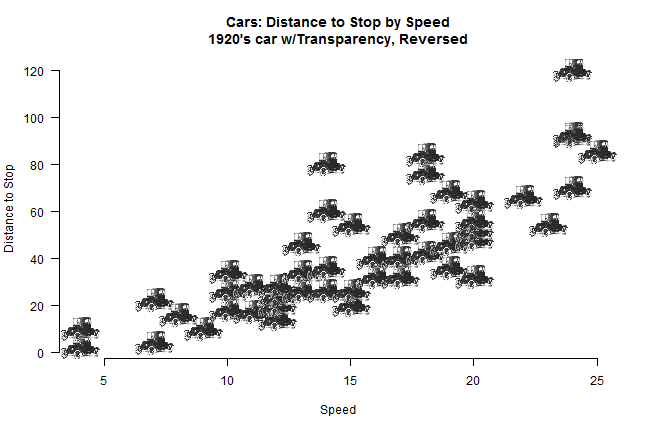## Tuesday, November 5, 2013

### Spider Web Plots in R

**Edit** I found a similar plotting technique using radarchart from the fmsb package, found at http://artax.karlin.mff.cuni.cz/r-help/library/fmsb/html/radarchart.html

Unless your brain works differently than mine, then polar coordinates aren't great for being precise in consuming and measuring data. Use bar charts, not pie charts, right angles! That being said, “spider plots” or “web plots” or whatever you want to call these charts, can succinctly summarize a lot of information, and are visually pleasing.

Here's a function to create this kind of plot.


webplot = function(data, data.row = NULL, y.cols = NULL, main = NULL, add = F,
col = "red", lty = 1, scale = T) {
if (!is.matrix(data) & !is.data.frame(data))
stop("Requires matrix or data.frame")
if (is.null(y.cols))
y.cols = colnames(data)[sapply(data, is.numeric)]
if (sum(!sapply(data[, y.cols], is.numeric)) > 0) {
out = paste0("\"", colnames(data)[!sapply(data, is.numeric)], "\"",
collapse = ", ")
stop(paste0("All y.cols must be numeric\n", out, " are not numeric"))
}
if (is.null(data.row))
data.row = 1
if (is.character(data.row))
if (data.row %in% rownames(data)) {
data.row = which(rownames(data) == data.row)
} else {
stop("Invalid value for data.row:\nMust be a valid rownames(data) or row-index value")
}
if (is.null(main))
main = rownames(data)[data.row]
if (scale == T) {
data = scale(data[, y.cols])
data = apply(data, 2, function(x) x/max(abs(x)))
}
data = as.data.frame(data)
n.y = length(y.cols)
polar.vals = (90 + seq(0, 360, length.out = n.y + 1)) * pi/180

#
plot(0, xlim = c(-2.2, 2.2), ylim = c(-2.2, 2.2), type = "n", axes = F,
xlab = "", ylab = "")
title(main)
lapply(polar.vals, function(x) lines(c(0, 2 * cos(x)), c(0, 2 * sin(x))))
lapply(1:n.y, function(x) text(2.15 * cos(polar.vals[x]), 2.15 * sin(polar.vals[x]),
y.cols[x], cex = 0.8))

lapply(seq(0.5, 2, 0.5), function(x) lines(x * cos(seq(0, 2 * pi, length.out = 100)),
x * sin(seq(0, 2 * pi, length.out = 100)), lwd = 0.5, lty = 2, col = "gray60"))
lines(cos(seq(0, 2 * pi, length.out = 100)), sin(seq(0, 2 * pi, length.out = 100)),
lwd = 1.2, col = "gray50")
}

r = 1 + data[data.row, y.cols]
xs = r * cos(polar.vals)
ys = r * sin(polar.vals)
xs = c(xs, xs)
ys = c(ys, ys)

lines(xs, ys, col = col, lwd = 2, lty = lty)

}


Using the mtcars data set:

webplot(mtcars)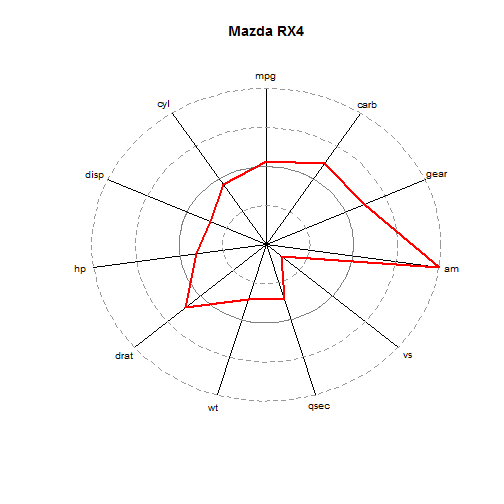Basically, we take a data frame, scale all numeric columns, and then plot how far above/below average (the thick black line) each of the values are for a particular row of data.

Here are the Arguments:

• data - data.frame or matrix
• data.row - row of data to plot (if NULL uses row 1)
• y.cols - columns of interest (if NULL it selects all numeric columns)
• main - title of plot (if NULL then rowname of data)
• add - whether the plot should be added to an existing plot
• col - color of the data line
• lty - lty of the data line

For example, we can change the data row that we plot, as well as the columns we want to include

webplot(mtcars, 2, y.cols = c("mpg", "cyl", "disp", "hp"))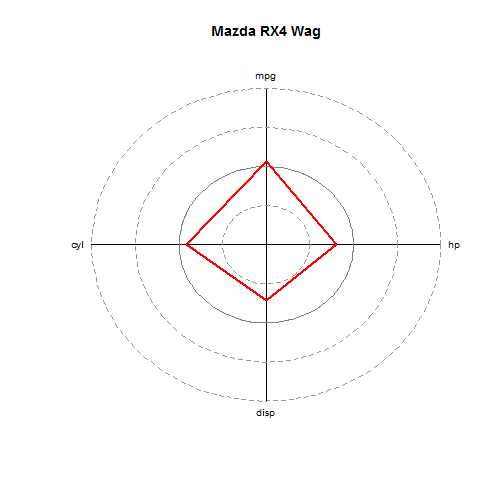It turns out that this isn't the easiest way to compare results, it might be helpful to overlay plots

par(mfcol = c(1, 2))
webplot(mtcars, "Mazda RX4")
webplot(mtcars, "Mazda RX4 Wag")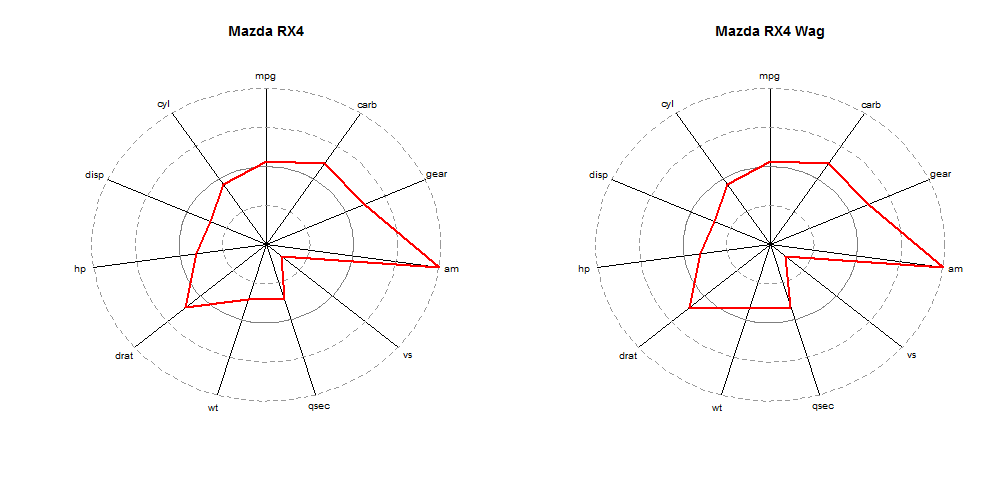par(mfcol = c(1, 1))

par(mar = c(1, 1, 2, 1))
webplot(mtcars, "Mazda RX4", main = "Compare Cars")
webplot(mtcars, "Mazda RX4 Wag", add = T, col = "blue", lty = 2)
par(new = T)
par(mar = c(0, 0, 0, 0))
plot(0, type = "n", axes = F)
legend("bottomright", lty = c(1, 2), lwd = 2, col = c("red", "blue"), c("Mazda RX4",
"Mazda RX4 Wag"), bty = "n")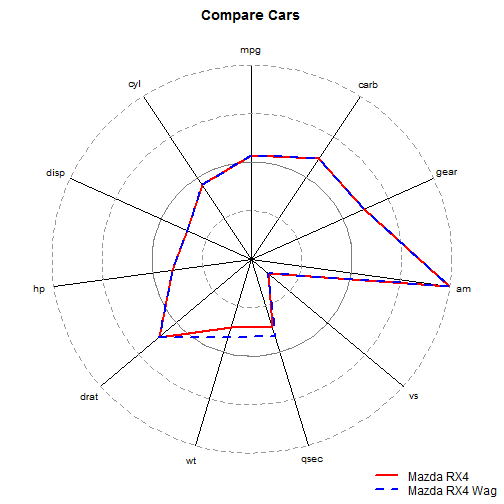Which makes it easy to observe that the Mazda RX4 and Mazda RX4 Wagon are very similar, with the Wagon being a little heavier, and takes a little longer to travel a quarter mile

## Thursday, September 19, 2013

### Using Custom Images as "pch" values in R

In a previous post I show how one can use custom pch values to represent different data types. I've long wanted to be able to take a custom image and use that to represent the data. I recently discovered the rasterImage function in R, which allows the user to take an image and plot it! There are various examples out there of using RasterImage (such as RJournal_2011-1_Murrell.pdf), but I didn't find anything that did exactly what I wanted it to do

the tricky part is that rasterImage requires you to define the 4 boundaries of the image. The goal is then to be able to define the boundaries by only specifying an x, y coordinate, and have code smart enough to display the image with the appropriate dimensions

Here are the required packages
library(RCurl)  #for reading file from a URL

## Loading required package: bitops

library(png)  #for reading in a .png, use library(jpeg) for a .jpg

The function I wrote takes the image, the (x,y) coordinates of where you want to plot your image, and for now a cex and a pos argument. I intend on expanding the options, and hopefully will improve these functionalities over time.

image_points = function(image, x, y, cex = 1, pos = NULL) {
if (length(x) != length(y)) {
}
dim.x = dim(image)  #image width
dim.y = dim(image)  #image height
if (dim.x == dim.y) {
# obtian the ratio of width to height or height to width
ratio.x = ratio.y = 1
} else if (dim.x < dim.y) {
ratio.x = dim.x/dim.y
ratio.y = 1
} else {
ratio.x = 1
ratio.y = dim.y/dim.x
}
cex = cex/10  #how large the image should be, divided by 10 so that it matches more closely to plotting points
pin = par()$pin #pin provides the width and height of the _active graphic device_ pin.ratio = pin/max(pin) #take the ratio usr = par()$usr  #usr provides the lower.x, lower.y, upper.x, upper.y values of the plotable region

# combine the active device dimensions, the image dimensions, and the
# desired output size
image.size.y = (usr - usr) * pin.ratio * cex
image.size.x = (usr - usr) * pin.ratio * cex
for (i in 1:length(x)) {
# plot each point pos can be NULL (default) or 1, 2, 3, or 4, corresponding
# to centered (defualt), bottom, left, top, right, respectively.
if (is.null(pos)) {
# centered at (x,y), define the bottom/top and left/right boundaries of the
# image
x.pos = c(x[i] - (image.size.x * ratio.x)/2, x[i] + (image.size.x *
ratio.x)/2)
y.pos = c(y[i] - (image.size.y * ratio.y)/2, y[i] + (image.size.y *
ratio.y)/2)

rasterImage(image, x.pos, y.pos, x.pos, y.pos)
} else if (pos == 1) {
x.pos = c(x[i] - (image.size.x * ratio.x)/2, x[i] + (image.size.x *
ratio.x)/2)
y.pos = c(y[i] - (image.size.y * ratio.y), y[i])
} else if (pos == 2) {
x.pos = c(x[i] - (image.size.x * ratio.x), x[i])
y.pos = c(y[i] - (image.size.y * ratio.y)/2, y[i] + (image.size.y *
ratio.y)/2)
} else if (pos == 3) {
x.pos = c(x[i] - (image.size.x * ratio.x)/2, x[i] + (image.size.x *
ratio.x)/2)
y.pos = c(y[i], y[i] + (image.size.y * ratio.y))
} else if (pos == 4) {
x.pos = c(x[i], x[i] + (image.size.x * ratio.x))
y.pos = c(y[i] - (image.size.y * ratio.y)/2, y[i] + (image.size.y *
ratio.y)/2)
}

rasterImage(image, x.pos, y.pos, x.pos, y.pos)  #plot image
}
}

I pulled an image from sweetclipart.com using getURLContent() and readPNG()

URL = ("http://sweetclipart.com/multisite/sweetclipart/files/imagecache/middle/sports_car_2_red.png")  #where the image is located
image = readPNG(getURLContent(URL))  #gets the content of the URL

image is an array of dimensions width $$\times$$ height $$\times$$ channels. The first 3 channels represent the R, G, and B values (scaled 0 to 1) of each pixel, with the 4th channel representing the alpha value (scaled 0 to 1, 0 representing transparent)
Only pngs have the alpha channel, so jpegs will have 3 channels. Not all pngs have the 4th channel, if they do have the 4th channel the background won't necessarily be transparent. More on alpha values in a bit

dim(image)  #width, height, alpha

##  275 550   4


Using the cars dataset we can plot the distance it took to stop by speed

data(cars)
plot(cars, type = "n", axes = F, xlab = "Speed", ylab = "Distance to Stop",
main = "Cars: Distance to Stop by Speed")
axis(1)
axis(2, las = 2)
x = cars$speed y = cars$dist
image_points(image, x, y, 2)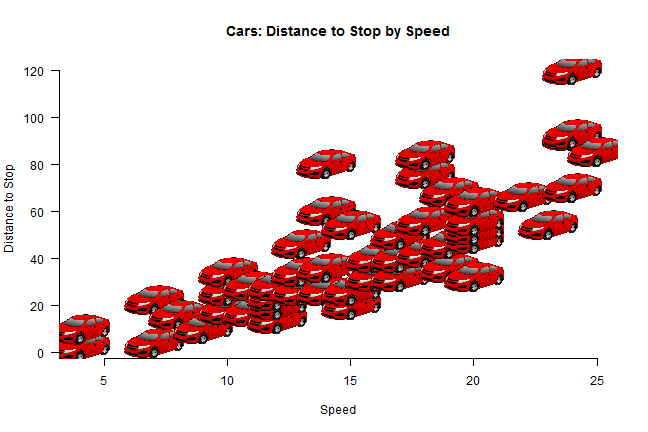It turns out that the data is from the 1920s, so using this image might be more appropriate:

library(jpeg)
URL2 = "http://embed.polyvoreimg.com/cgi/img-thing/size/y/tid/4985430.jpg"
plot(cars, type = "n", axes = F, xlab = "Speed", ylab = "Distance to Stop",
main = "Cars: Distance to Stop by Speed\n1920's car")
axis(1)
axis(2, las = 2)
image_points(image2, x, y, 2)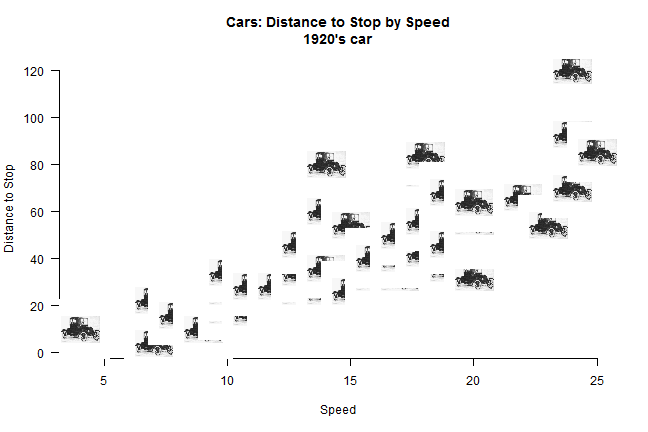Here we have a jpeg file that is a width $$\times$$ height matrix with values 0 to 1 representing the black/white/gray scale. We can manipulate the data using rgb() and abind() to create an array with the appropriate R, G, B, and alpha values.
First, on this color scale, values that are closer to 1 are going to be lighter gray to white, so we can assign an alpha value of 0 (transparent) to any values that are greater than 0.8.

alpha.val = matrix(1, nrow(image2), ncol(image2))  #want a matrix of 1s (not transparent) the same size as the image
alpha.val[image2 > 0.8] = 0  #for lighter gray and white values, set alpha to 0 (transparent)

We then want to create a new array, and will set the first dimension to the original values. Because it is gray colors, R=G=B

library(abind)
image2.adj = array(image2, dim = c(nrow(image2), ncol(image2), 1))

We can then plot it:

plot(cars, type = "n", axes = F, xlab = "Speed", ylab = "Distance to Stop",
main = "Cars: Distance to Stop by Speed\n1920's Car w/Transparency")
axis(1)
axis(2, las = 2)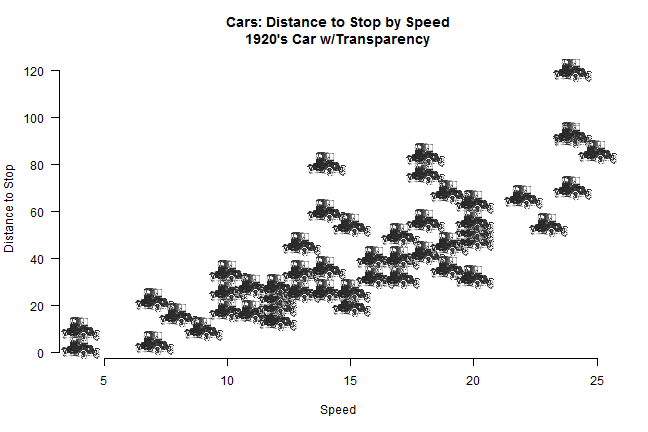Notice that we have now essentially removed the border around the image, and even though the images overlap, we get a better sense of whwere the points lie.

#### A few final notes

• If we want the cars to be reversed, we can simply…
image2.adj.reversed = image2.adj[, ncol(image2.adj):1, ]
plot(cars, type = "n", axes = F, xlab = "Speed", ylab = "Distance to Stop",
main = "Cars: Distance to Stop by Speed\n1920's car w/Transparency, Reversed")
axis(1)
axis(2, las = 2)